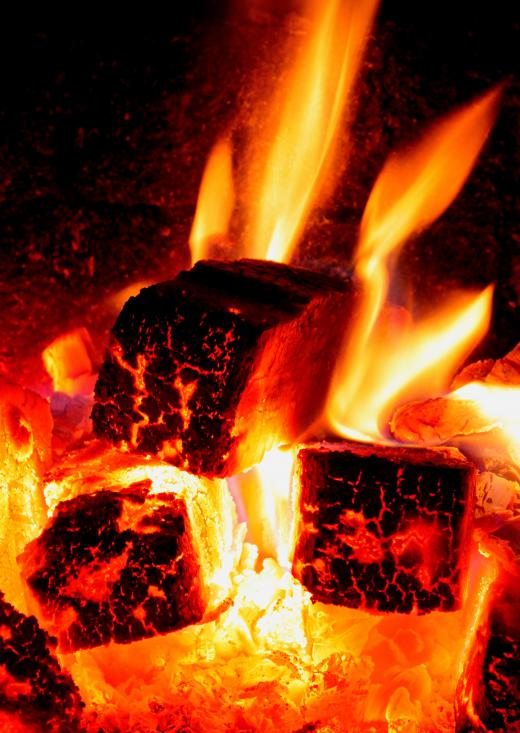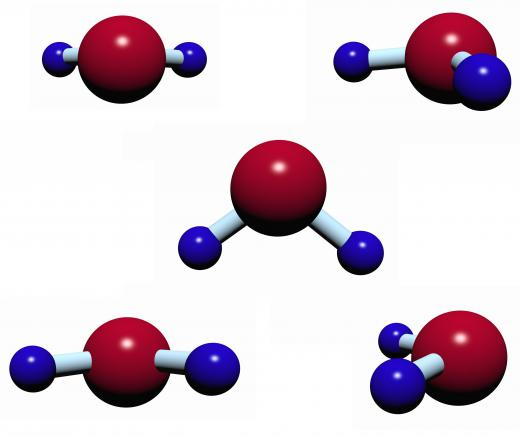# What is Specific Heat?

Daniel Liden
Daniel LidenKnowing the specific heat of a substance allows industries to calculate how much energy they will need to expend when operating a blast furnace or cracking tower.

Specific heat is a measurement used in thermodynamics and calorimetry that states the amount of heat energy necessary to increase the temperature of a given mass of a particular substance by some amount. While different scales of measurement are sometimes used, this term usually specifically refers to the amount required to raise 1 gram of some substance by 1.8°F (1° Celsius). It follows that if twice as much energy is added to a substance, its temperature should increase by twice as much. Specific heat is usually expressed in joules, the unit typically used in chemistry and physics to describe energy. It is an important factor in science, engineering, and in understanding the Earth’s climate.

#### Heat and TemperatureSpecific heat is the amount of energy required to increase the temperature of a given mass of a specific substance.

Heat energy and temperature are two different concepts, and understanding the difference is important. The first is a quantity in thermodynamics that describes the amount of change that a system can cause to its environment. The transfer of this energy to an object causes its molecules to move around more rapidly; this increase in kinetic energy is what is measured, or experienced, as an increase in temperature.

#### Specific Heat and Heat CapacityIts molecular structure gives water a very high specific heat.

These two properties are often confused. The first is the number of joules required to increase the temperature of a given mass of a substance by some unit. It is always given “per unit mass,” for example, 0.45 j/g°C, which is the specific heat of iron, or the number of joules of heat energy to raise the temperature of one gram of iron by one degree Celsius. This value is therefore independent of the amount of iron.

Heat capacity — sometimes called “thermal mass” — is the number of joules required to raise the temperature of a particular mass of material by 1.8°F (1°Celsius), and is simply the specific heat of the material multiplied by its mass. It is measured in joules per °C. The heat capacity of an object made of iron, and weighing 100g, would be 0.45 X 100, giving 45j/°C. This property can be regarded as the capacity of an object to store heat.

The specific heat of a substance holds more or less true over a wide range of temperatures, that is, the energy required to produce a one degree rise in a given substance varies only slightly with its initial value. It does not apply, however, when the substance undergoes a change of state. For example, if heat is continually applied to a quantity of water, it will produce a rise in temperature in accordance with water’s specific heat. When the boiling point is reached, however, there will be no further increase; instead, the energy will go into producing water vapor. The same applies to solids when the melting point is reached.

A now outdated measure of energy, the calorie, is based on the specific heat of water. One calorie is the amount of energy required to raise the temperature of one gram of water by 1.8°F (1°C) at normal air pressure. It is equivalent to 4.184 joules. Slightly different values may be given for the specific heat of water, as it varies a little with temperature and pressure.

#### Effects

Different substances can have very different specific heats. Metals, for example, tend to have very low values. This means that they heat up quickly and cool down quickly; they also tend to expand significantly as they get hotter. This has implications for engineering and design: allowance often has to be made for the expansion of metal parts in structures and machinery.

Water, in contrast, has a very high specific heat — nine times that of iron, and 32 times that of gold. Due to the molecular structure of water, a great deal of energy is needed to increase its temperature by even a small amount. It also means that warm water takes a long time to cool down.

This property is essential to life on Earth, as water has a significant stabilizing effect on the global climate. During the winter, oceans cool down slowly and release a significant amount of heat into the environment, which helps keep the global temperature reasonably stable. Conversely, in summer, it takes a great deal of heat to significantly increase ocean temperatures. This has a moderating effect on climate. Continental interiors, far from the ocean, experience far greater extremes of temperature than coastal regions.anon354488

I would like to ask a question about heat. We all know in the real world that melting the same mass of bronze is much harder than melting aluminum. So it means to melt bronze I will need much more heat than to melt aluminum

if this is true. Why is the specific heat of aluminum (.215 BTU/LB-F)

higher than bronze (0.104 BTU/LB-F)?

anon297407

If energy is transferred to a substance as heat, will the temperature of the substance always increase -- why or why not?

aplenty

@ Istria- Specific heat is an important value that is used to determine the amount of energy transferred between a system and its surroundings.

Let's say you wanted to determine the amount of energy transferred from a 50g block of aluminum heated to 100 degrees Celsius dropped in a container filled with water. You would use the specific heat of aluminum (0.900J/gC) the mass of the aluminum (50g) and the change in temperature (let's say it was 10C in this case).

You could plug these numbers into the specific heat equation (q=Cs∙m∙∆T) and you would know that the aluminum lost 450 Joules of energy. Conversely, the first law of thermodynamics would tell you that the water gained 450 Joules of energy because energy is constant so it must travel from a system to its surroundings.

istria

How are specific heat and energy relevant? What is the importance of knowing the specific heat, and what is specific heat useful in determining? I am just curious.

highlighter

I just want to ad that the unit for specific heat is Joules per gram Kelvin, or more casually Joules per gram Celsius. If you were to simply tell someone a number without specifying Joules per grams Kelvin, then you would cause confusion. Joules refers to energy.

The specific heat of an object is an intensive property of an element, meaning that it does not depend on the sample size. An intensive property will always be constant regardless of the amount of a substance, element, etc. Energy on the other hand is an extensive property or variable because it is dependent on the mass, volume, distance traveled, etc. of an element or object. There is a big difference between the two, and interchanging the units in a specific heat calculation can misconstrue meaning or lead to miscalculations.